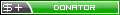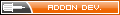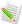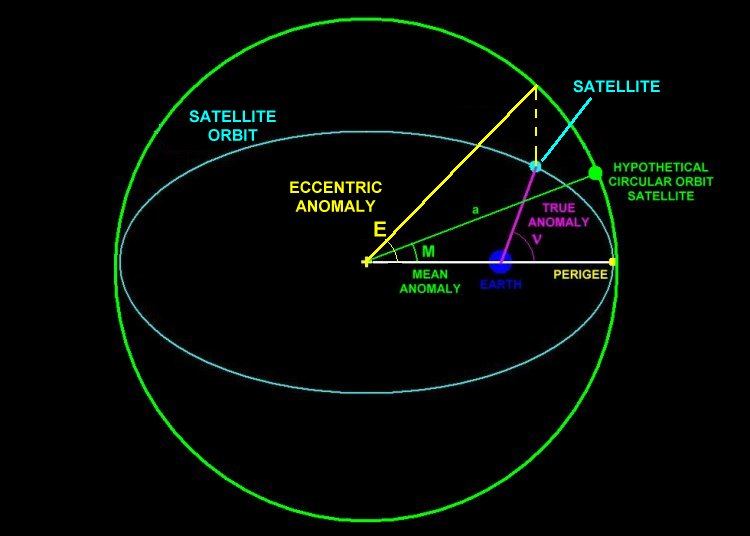Orbiter-ForumSo, you want to calculate orbits?
 User Name Remember Me? Password
 Register Blogs Orbinauts List Social Groups FAQ Projects Mark Forums Read

#1
 george7378DON'T PANICSo, you want to calculate orbits?
by george7378 03-04-2012, 02:09 PM

Well, do you? If so, I hope this can show you how to calculate and therefore simulate an orbit yourself! By this I mean you will be able to calculate a satellite's altitude and velocity at a chosen time, among other things, like OrbitMFD shows. Let's assume we're orbiting Earth, and that there are no other forces acting on the satellite other than the Earth's gravity. So, what do we do with this assumption?

1. Decide on an apogee and perigee.

Let's have a nice sensible 400 x 1400 km orbit.

2. Calculate some constants for the orbit.

These constants are a)Apogee and perigee radii b) Semi-major axis, c) Eccentricity, d) Mean motion and e) Orbital period.

a) Apogee and perigee radii are simply the apogee and perigee + the radius of Earth:= 7778km= 6778km

b) Semi-major axis is the distance from the centre of your orbit to one of the extreme ends:= 7278km

c) Eccentricity is how close (or far away, depending on how you look at it) your orbit is to being a circle:= 0.069

d) Mean motion is a measure of how quickly a satellite moves in its orbit (The constant GM is the gravitational constant multiplied by Earth's mass, and equals 398600.4):= 1.02 x 10^-3

e) Orbital period is how long it takes to complete one orbit:= 6179 seconds

3. Decide on a time for which you want to calculate the altitude and velocity.

By 'a time', I mean 'how far into the orbit do you want to be?' - eg: if t=0, you are at perigee. If t=T/2, you are halfway round the orbit and at apogee. If you choose anything in between, you will calculate for that position. So this basically means 'how many seconds past the perigee do you want to be?' - you can't have a negative number, or a number larger than the orbital period.

We will calculate for the apogee. Therefore:

t = T/2 = 3089.5 seconds.

4. Calculate some anomalies (angles).

These are simply angles between different points in the orbit. They are a) Mean anomaly, b) Eccentric anomaly and c) True anomaly. To understand them, it's best to see a diagram like this one:These angles are in radians!

a) Mean anomaly:= 3.15

b) To find the Eccentric anomaly, you have to iterate. The formula you need to iterate is:To start, makeIf you're not faimilar with iteration, it means that you have to take an initial value (in this case, M, your Mean anomaly) and substitute it into the given equation. Then, you replace your initial value with your result of your first calculation, and do the same thing again and again until the result you get doesn't change. In this case, because you are at apogee, E = M, so you get:

E = 3.15

c) True anomaly:= 3.15

As you can see, all three anomalies are equal at this point, meaning that our method is working (since we are calculating for the apogee where they all ~ 3.15 radians. The actual value is 3.141 radians, but the reason we are slightly out is because of rounding in our previous answers. No biggie at all).

5. Calculate the final result!

To arrive at the final result of altitude and velocity, you need one more interim value. The flightpath:= -6.2 x 10^-4

A little note: This number should be zero at apogee, but again, it's because of rounding. This is not a big deal, and will still give us a perfectly accurate answer.

Finally, we can now calculate our results!= 1402km.= 6.91km/s

As you can see, there are minor errors of ~ 2km in altitude, and hence a tiny error in velocity, but this is all because of rounding in the constants and prior results. If you use a program to solve these equations, you will get far more accurate values.

It is of course inconvenient to solve these equations manually, but if used as part of a program which continues to solve as time increases, a very accurate orbit simulation can be made!

I have created a program which runs on these equations alone, and which simulates an Earth-orbiting satellite in the way we just have. It's called Orbit-Vis, and is available here:

http://sourceforge.net/projects/orbitvis/

Views 57008 Comments 0
 Thanked by:

 Article ToolsShow Printable VersionEmail this PagePosting Rules BB code is On Smilies are On [IMG] code is On HTML code is Off You may not post new threads You may not post replies You may not post attachments You may not edit your posts
 Forum Jump User Control Panel Private Messages Subscriptions Who's Online Search Forums Forums Home Orbiter-Forum.com     Announcements     Meets & Greets Orbiter Space Flight Simulator     Orbiter Web Forum         OFMM         Orbiter Forum Space Station         Simpit Forum     General Questions & Help     MFD Questions & Help     Hardware & Software Help     Tutorials & Challenges     Orbiter SDK     Orbiter Visualization Project     Orbiter Beta » Orbiter Project Orbiter Addons     OrbitHangar Addons & Comments     Addons     Addon Development     Addon Requests     Addon Support & Bugs         Addon Developer Forums             Project Apollo - NASSP     Orbiter Lua Scripting Far Side of the Moon     Spaceflight News     Math & Physics     Astronomy & the Night Sky     Backyard Rocketry     Brighton Lounge     International Forum

All times are GMT. The time now is 02:43 AM.

About Us | Rules & Guidelines | TOS Policy | Privacy Policy

Orbiter-Forum is hosted at Orbithangar.com